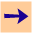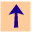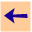3 Syntax

# 3 Syntax

The syntax of logical terms is based on Prolog's syntax in its convention for variables, but uses a different syntax for operators (because Prolog's are so confusing and to emphasize it is not Prolog).

A variable is a sequence of alphanumeric characters (possibly including "`_`") that starts with an upper case letter or "`_`". For example, `X`, `Letter`, `Any_cat`, `A_big_dog` are all variables. The variable "`_`" in an anonymous variable which means that all occurrences are assumed to be different variables. If a variable occurs only once in a clause, it should probably be written as "`_`".

A constant is either:

• a sequence of alphanumeric characters (possibly including "`_`") starting with a lower case letter, such as: `david`, `comp_intell`, `ciLOG`, and `a45_23`
• an integer or float, such as `123`, `-5`, `1.0`, `-3.14159`, `4.5E7`, `-0.12e+8`, and `12.0e-9`. There must be a decimal point in floats written with an exponent and at least one digit before and after a decimal point.
• any sequence of characters delimited by single quotes, such as `'X'`, `'2b~2b'`, `'../ch2/foo.pl'`, `'A Tall Person'`

A term is either a variable, a constant, of of the form f(t1,...,tn), where f, a function symbol, is a sequence of alphanumeric characters (possibly including "`_`") starting with a lower case letter and the ti are terms.

An atom is either of the form p or p(t1,...,tn), where p, a predicate symbol, is a sequence of alphanumeric characters (including `_`) starting with a lower case letter and the ti are terms.

An body is either an atom, of the form alpha`&`beta, where alpha and beta are bodies, or of the form `~`alpha, where alpha is a body.

A clause is either an atom or is a rule of the form h`<-`b where h is an atom (the head of the clause) and b is a body.

Some predicate and function symbols can be written using infix notation. For example "`X is 4+3*Y`" means the same as "`is(X,+(4,*(3,Y)))`", where "`is`" is a predicate symbol and "`+`" and "`*`" are function symbols. The operator precedence follows Prolog's conventions.

The symbol "`<=`" is defined to be infix, but there are no clauses defining it. This is designed to be used for meta-programming where "`<=`" can be used as a meta-level predicate symbol defining the object-level implication. (See Computational Intelligence , Chapter 6).3 Syntax